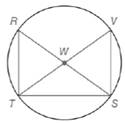Chapter 6.1, Problem 32EElementary Geometry For College St...

7th Edition
Alexander + 2 others
ISBN: 9781337614085

Solutions

Chapter
SectionElementary Geometry For College St...

7th Edition
Alexander + 2 others
ISBN: 9781337614085
Textbook Problem

In Exercises 32 to 37, write a paragraph proof. Given: R S ¯  and  T V ¯  are diameters of  ⊙ W Prove: Δ R S T ≅ Δ V T STo determine

To prove:

A paragraph proof when RS¯ and TV¯ are diameters of W then ΔRSTΔVTS.

Explanation

Given:

The line segments RS¯ and TV¯ are diameters of W.

Rule used:

SAS congruency rule:

If two sides and the included angle of one triangle are equal to two sides and included angle of another triangle, then the triangles are congruent.

SSS congruency rule:

If three sides of one triangle are equal to three sides of another triangle then the triangles are congruent.

Proof:

Let RS¯ and TV¯ be the diameters of circle W.

Since all the diameters of the circle are of same length, RS¯TV¯.

Also, all the radii of the circle are of same length, WR¯WV¯ and WT¯WS¯.

From the figure, we see that the diameters RS¯ and TV¯ form vertical angles, which are congruent

Still sussing out bartleby?

Check out a sample textbook solution.

See a sample solution

The Solution to Your Study Problems

Bartleby provides explanations to thousands of textbook problems written by our experts, many with advanced degrees!

Get Started

Prove the statement using the , definition of a limit. limx10(345x)=5

Single Variable Calculus: Early Transcendentals, Volume I

The length of the curve given by x = 3t2 + 2, y = 2t3, is:

Study Guide for Stewart's Single Variable Calculus: Early Transcendentals, 8th

Using a binomial series, the Maclaurin series for is:

Study Guide for Stewart's Multivariable Calculus, 8th